Recent advances in the understanding of the rapid neutron capture processLA-UR-19-XXXXX

Matthew Mumpower

ANL Seminar

Monday Sept. 9$^{th}$ 2019FIRE Collaboration
Fission In R-process Elements

To understand the formation of the elements

Requires deep knowledge of a range of fields, including:

The theoretical modeling of astrophysical environments

Multi-messenger observations (gravitational waves, EM waves, etc.)

Nuclear theory predictions for exotic nuclei

Precision experiments to constrain nuclear theory

Data and observations are limited

We must be clever when deciphering what is going on with nucleosynthesis...

What is the $r$-process?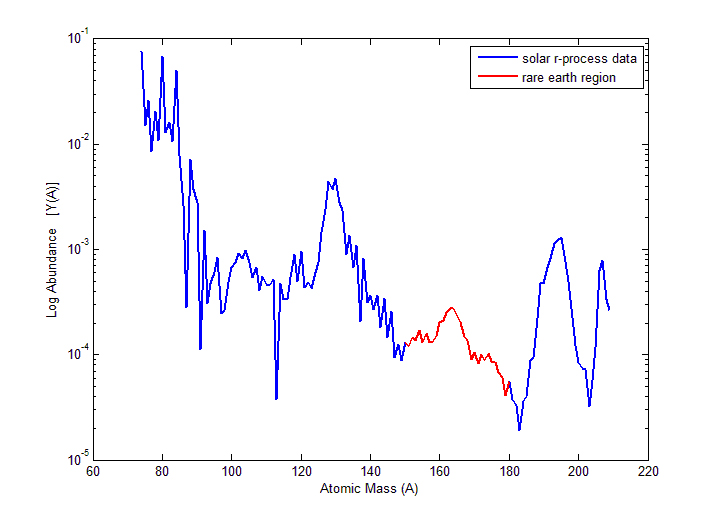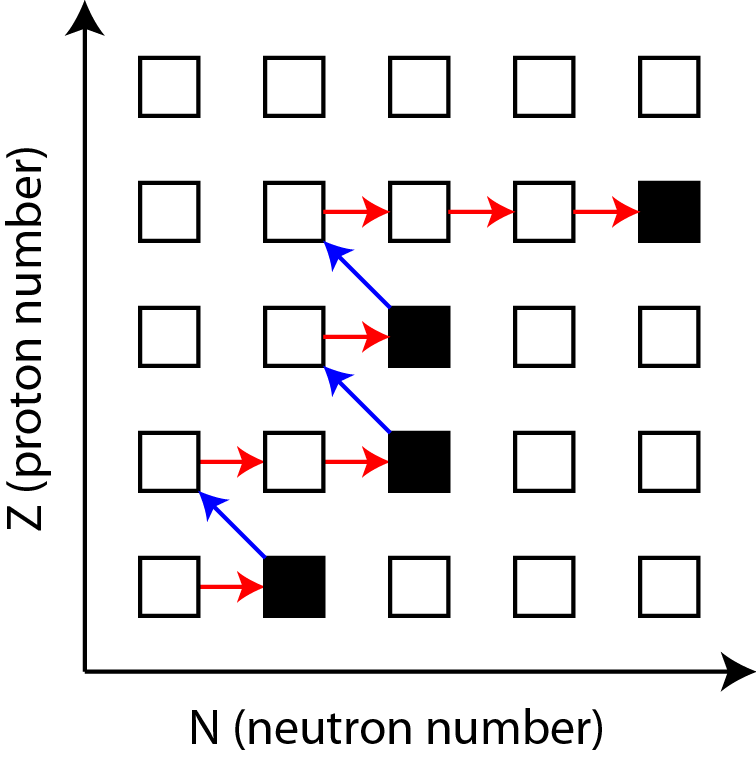Rapid neutron capture that occurs in astrophysical environments allowing for the production of heavy elements

Neutron captures are initially much faster than $\beta$-decays

Relative slowdown in the nuclear flow (right) produces peak structures in the observed abundances (left)

Astrophysical environment must produce a lot of free neutrons in order for this process to proceed

Aprahamian et al. (2018) • Horowitz et al. J Phys G 083001 (2019)

Where can the $r$-process occur?

One possibility is in (rare?) supernovae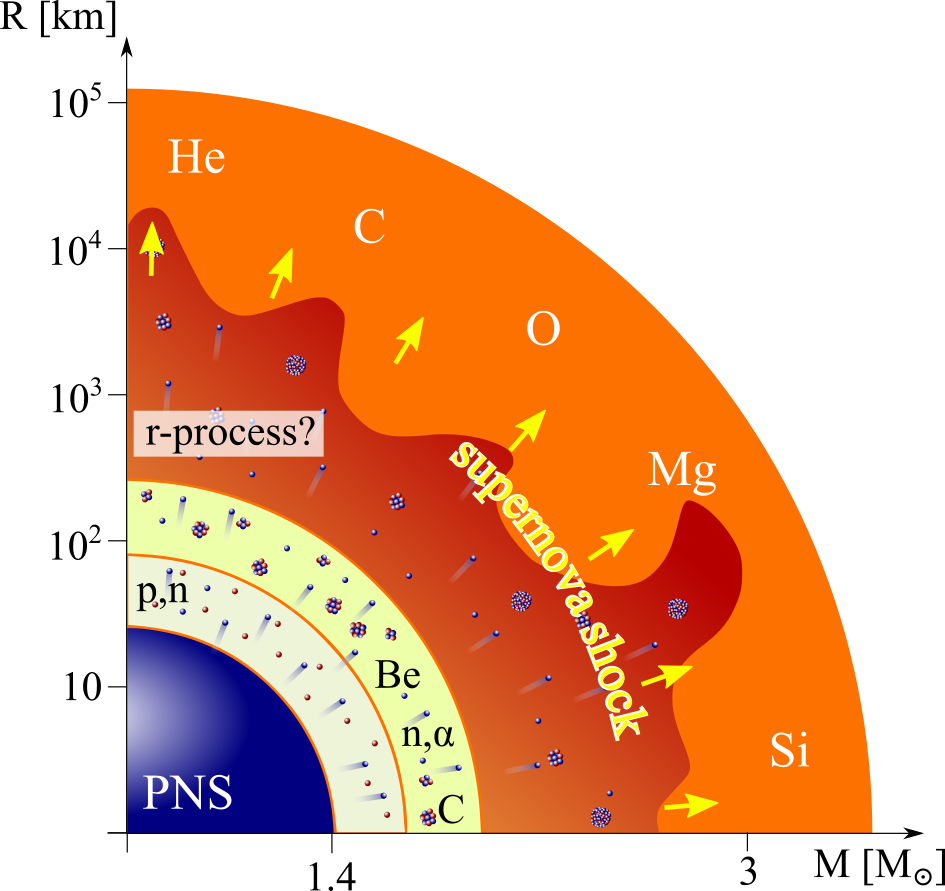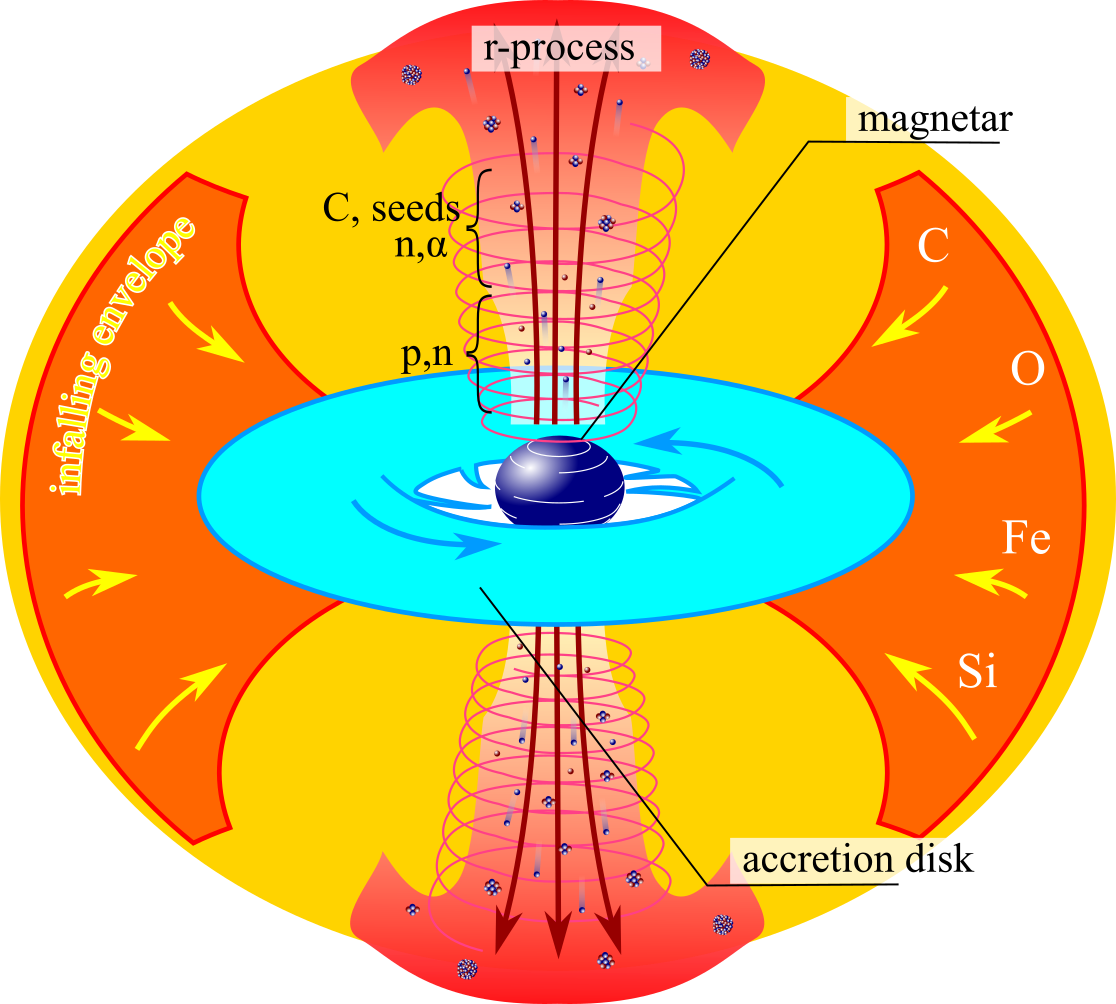For standard supernovae (left) neutrino physics still needs to be well understood

Jets in magnetorotational driven supernovae (right) may also provide the necessary conditions

Another option is the disk winds of collapsars - black hole forms after core collapse of a rapidly rotating star

Meyer ApJ (1989) • MacFadyen & Woosley ApJ (1999) • Figure from Mumpower et al. in prep. (2019)

Where can the $r$-process occur?

Another possibility is in compact object mergers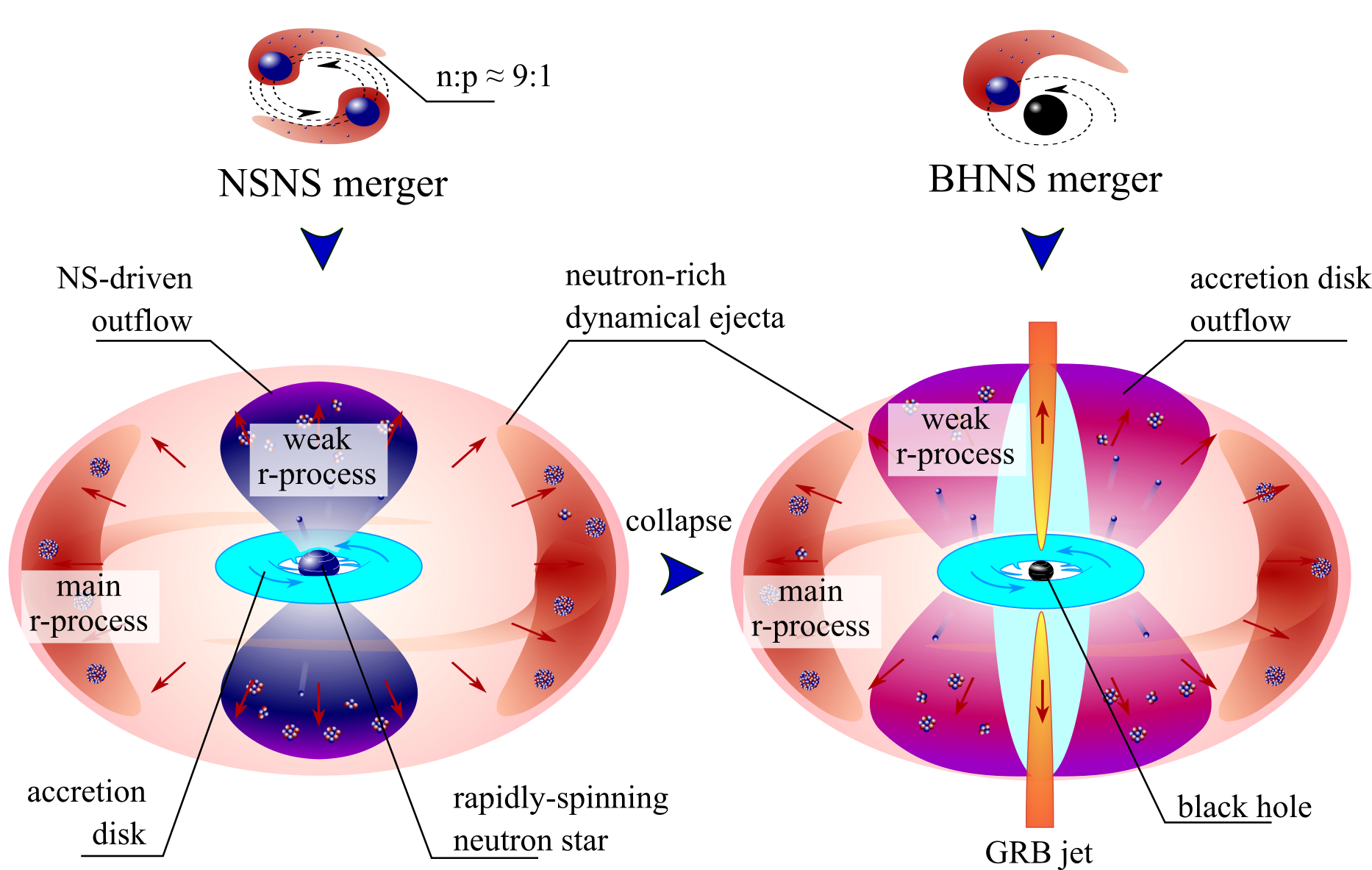A binary merger of neutron stars is an exciting possibility (some indirect evidence exists)

Another option is in the disk of a black hole neutron star binary

Lattimer & Schramm (1974) • Korobkin et al. (2012) • Figure from Mumpower et al. in prep. (2019)

How can we observe the $r$-process?

Gravitational waves are emitted by explosive events (can be detected by LIGO)

Electromagnetic signals may be detected from the radioactive decay of heavy nuclei

The problem with EM signals is that we can only detect them if the event is close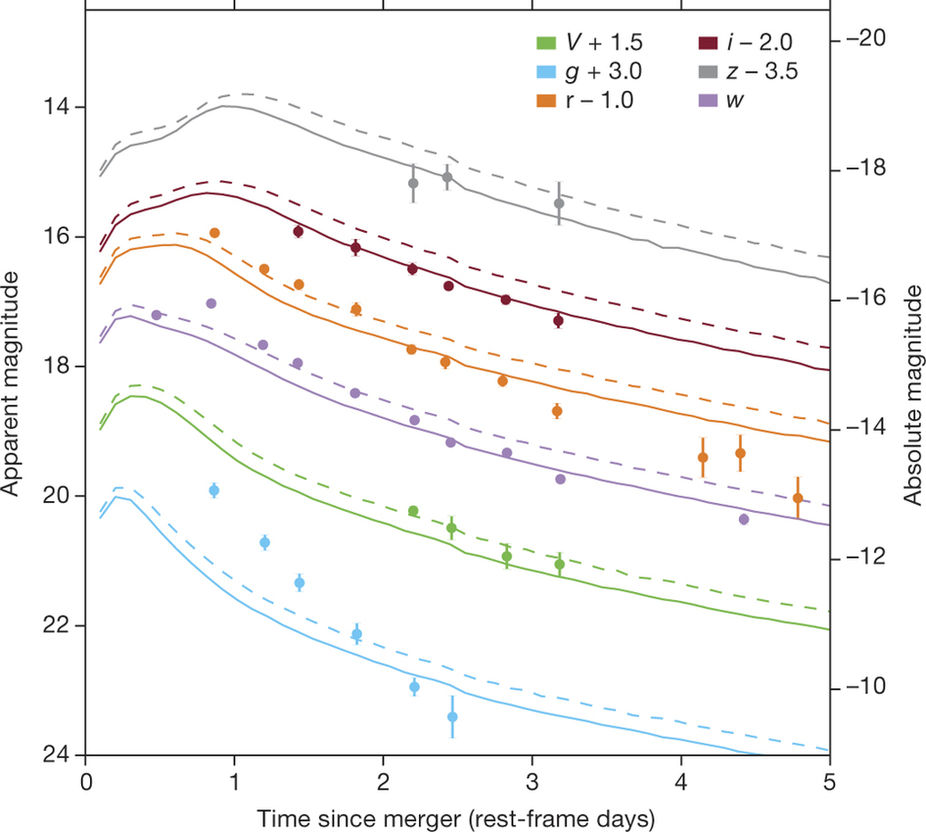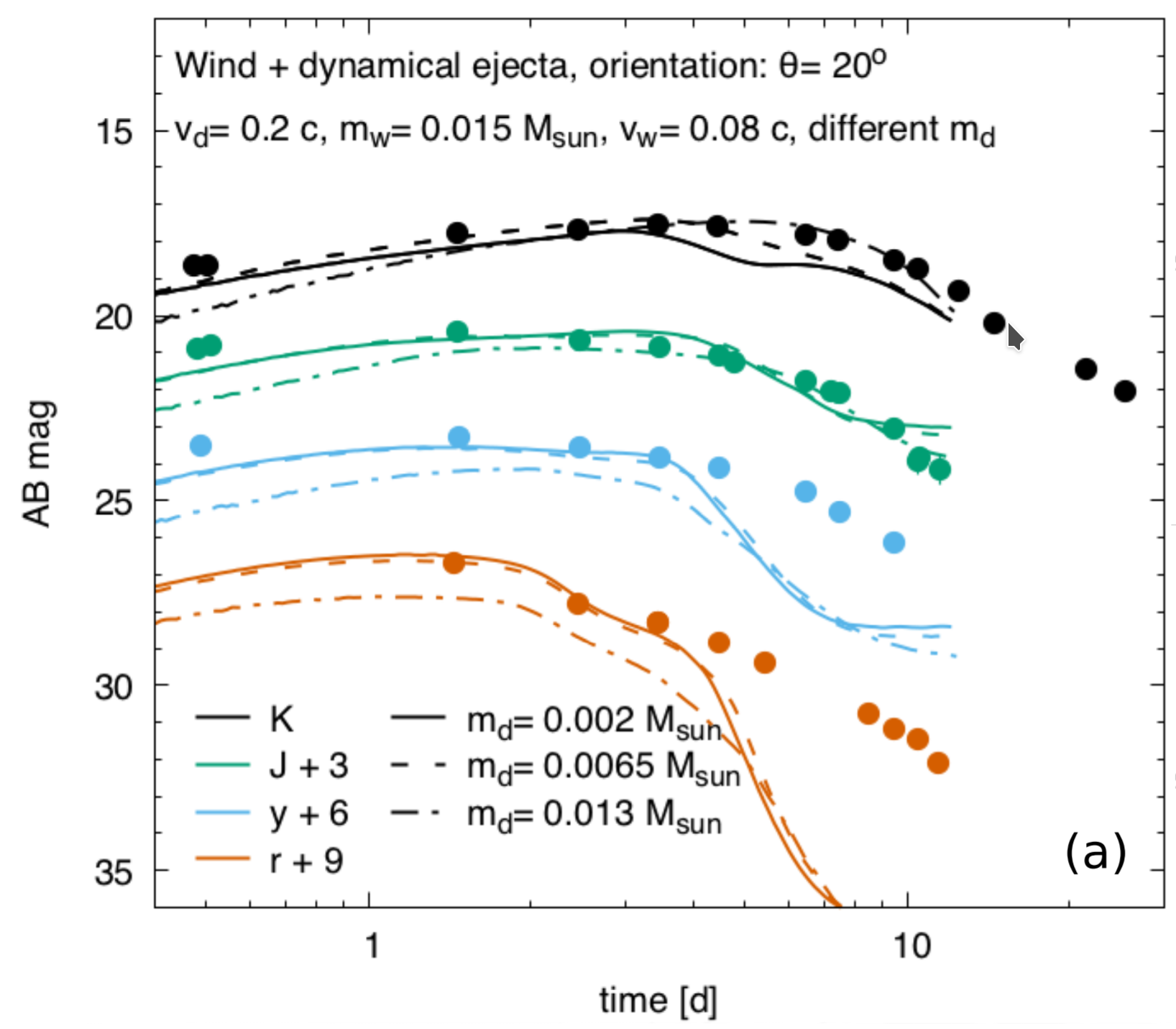(left) Low lanthanide fraction (right) high lanthanide fraction → major degeneracies in the model space

Arcavi et al. Nature 551 64 (2017) • Tanvir et al. ApJL 848 2 (2017)

Schematic of a kilonova (NSNS)

2 component model: wind & dynamical eject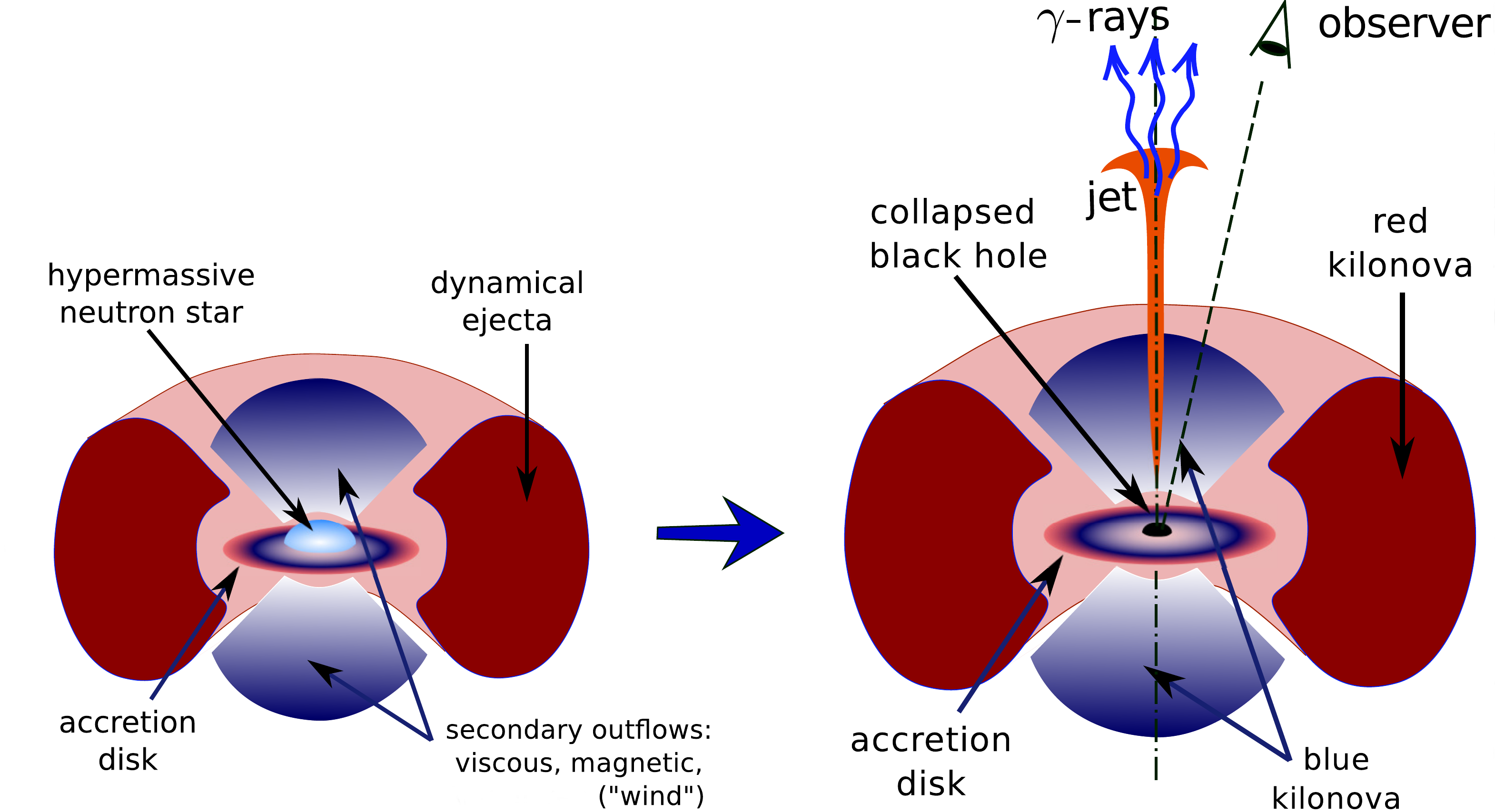Red emission: late (weeks); lanthanide dominated

blue emission: early (days); UV dominated

Metzger et al. MNRAS 406 4 (2010) • Kasen et al. Nature 551 80 (2017) • Figure by O. Korobkin

When we model nucleosynthesis

We want to describe the abundances observed in nature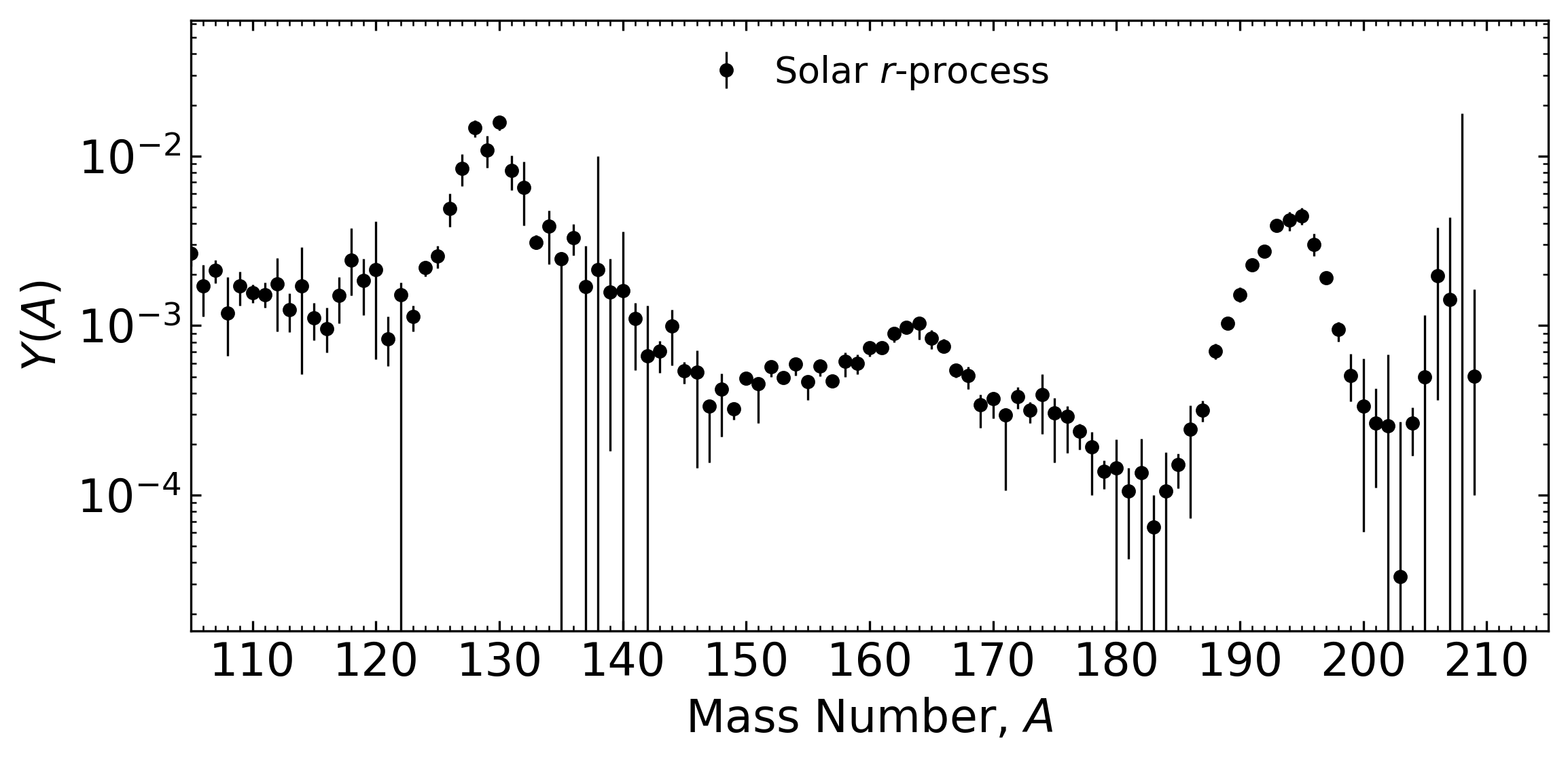But there is uncertainty in:

The astrophysical conditions (large variations in current simulations)

The nuclear physics inputs (1000's of unknown species / properties)

Mumpower et al. PRC (2012) • Mumpower et al. ApJ (2012) • Mumpower et al. PRC (2015)

Inputs from nuclear physics

1st order: masses, $\beta$-decay rates, reaction rates & branching ratios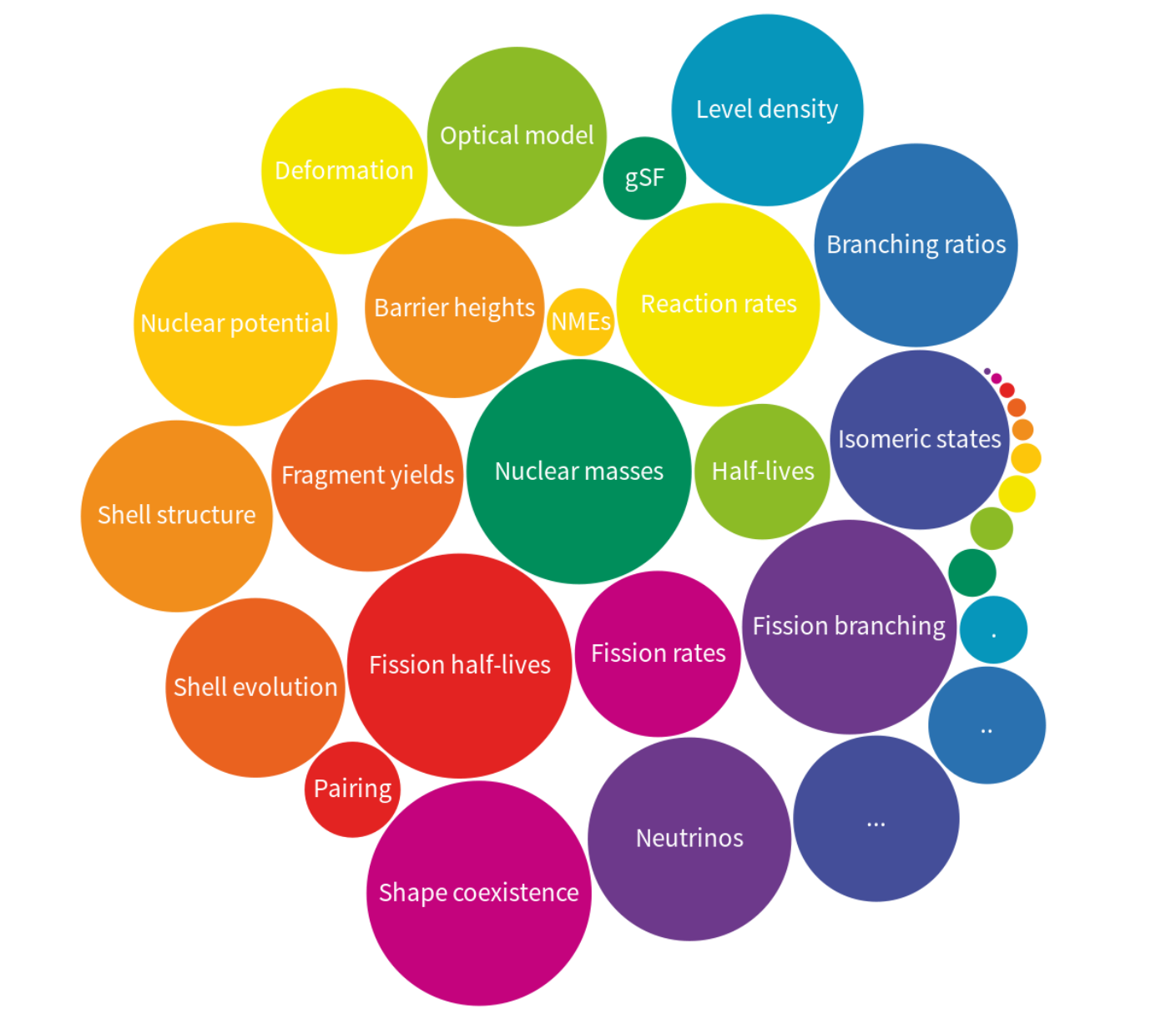See review paper: Mumpower et al. PPNP 86 (2016)

What do we know?

The chart of nuclides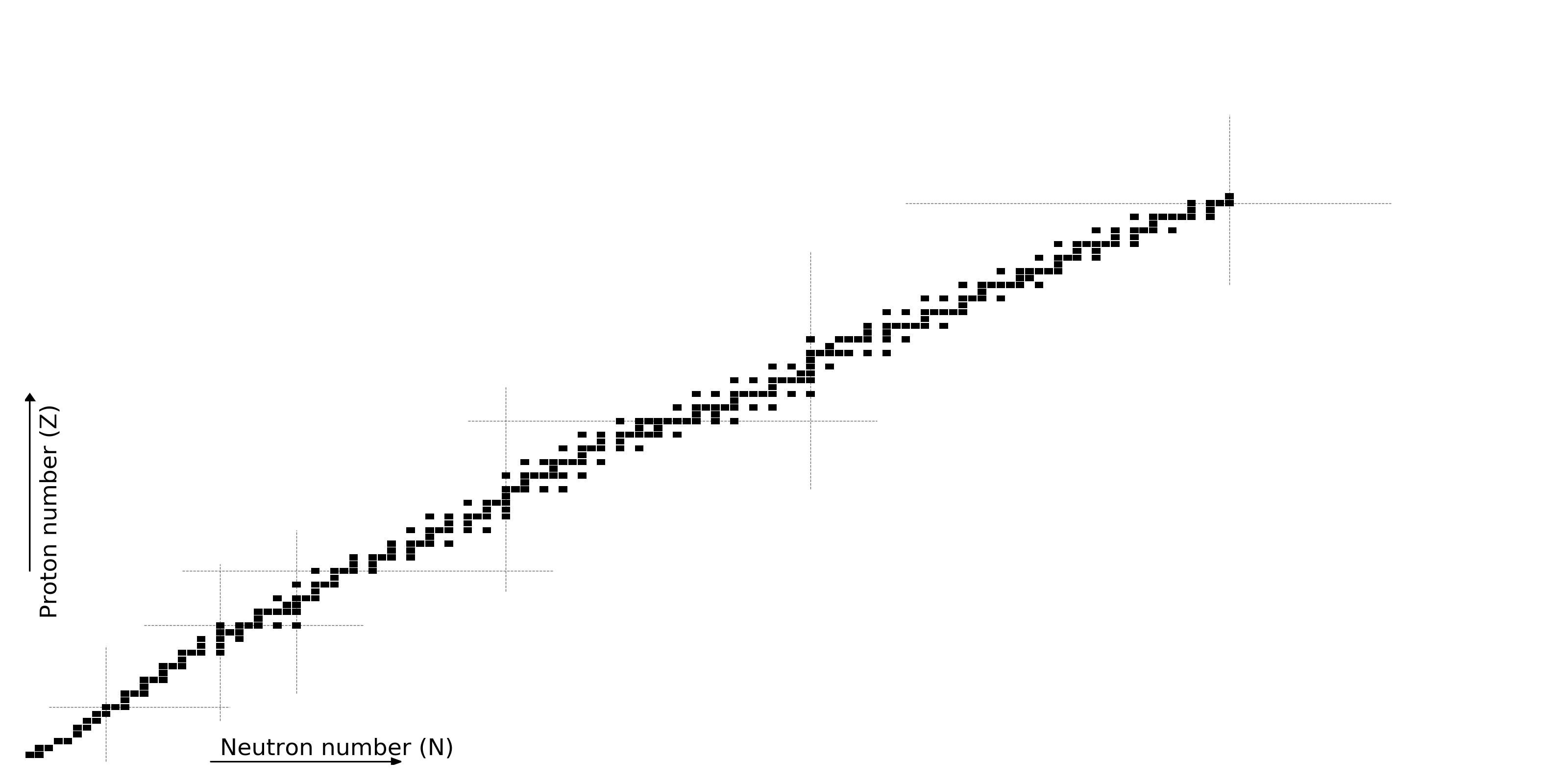Figure by Mumpower

What do we know?

All half-lives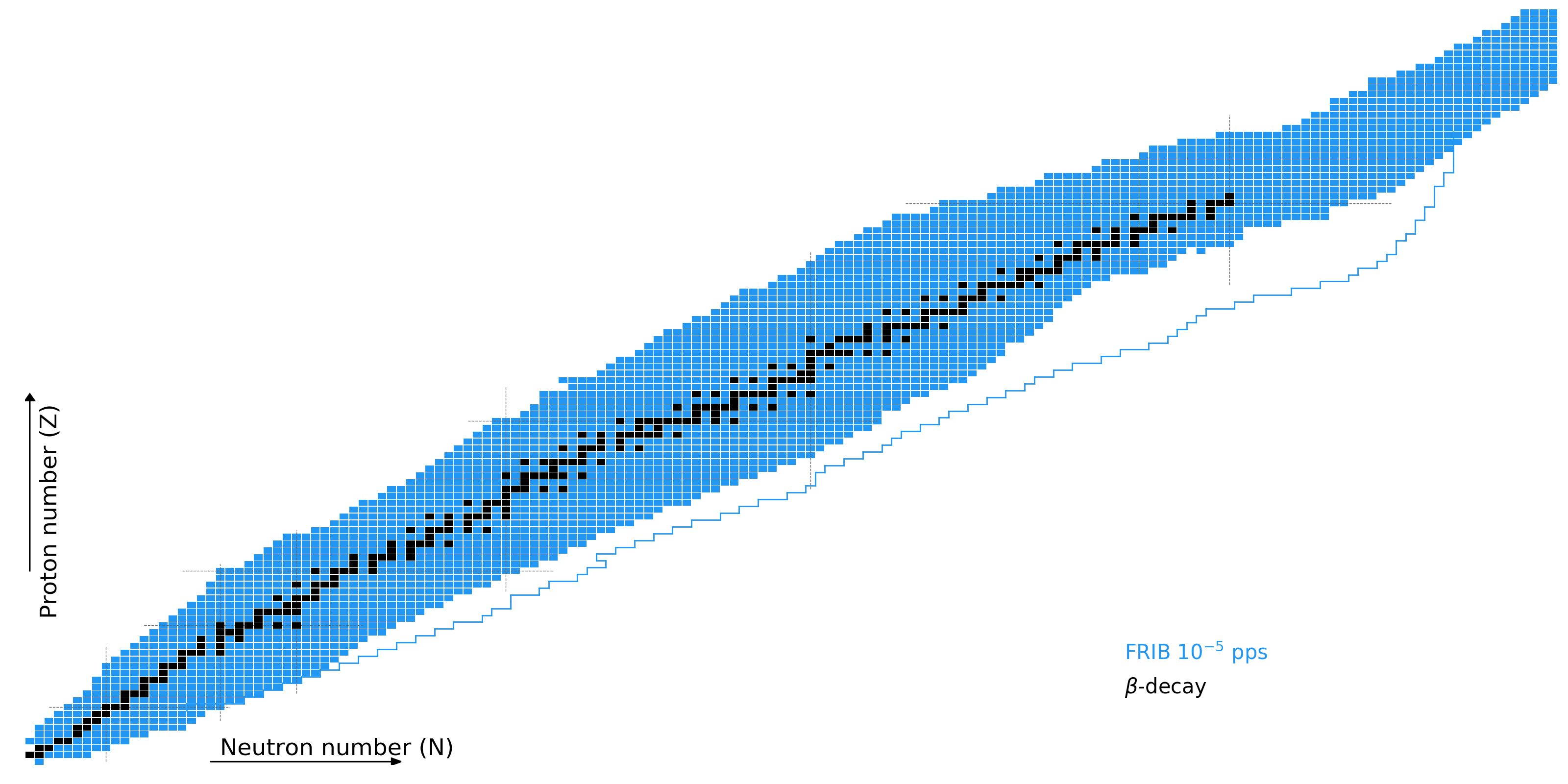Figure by Mumpower

What do we know?

Nuclear masses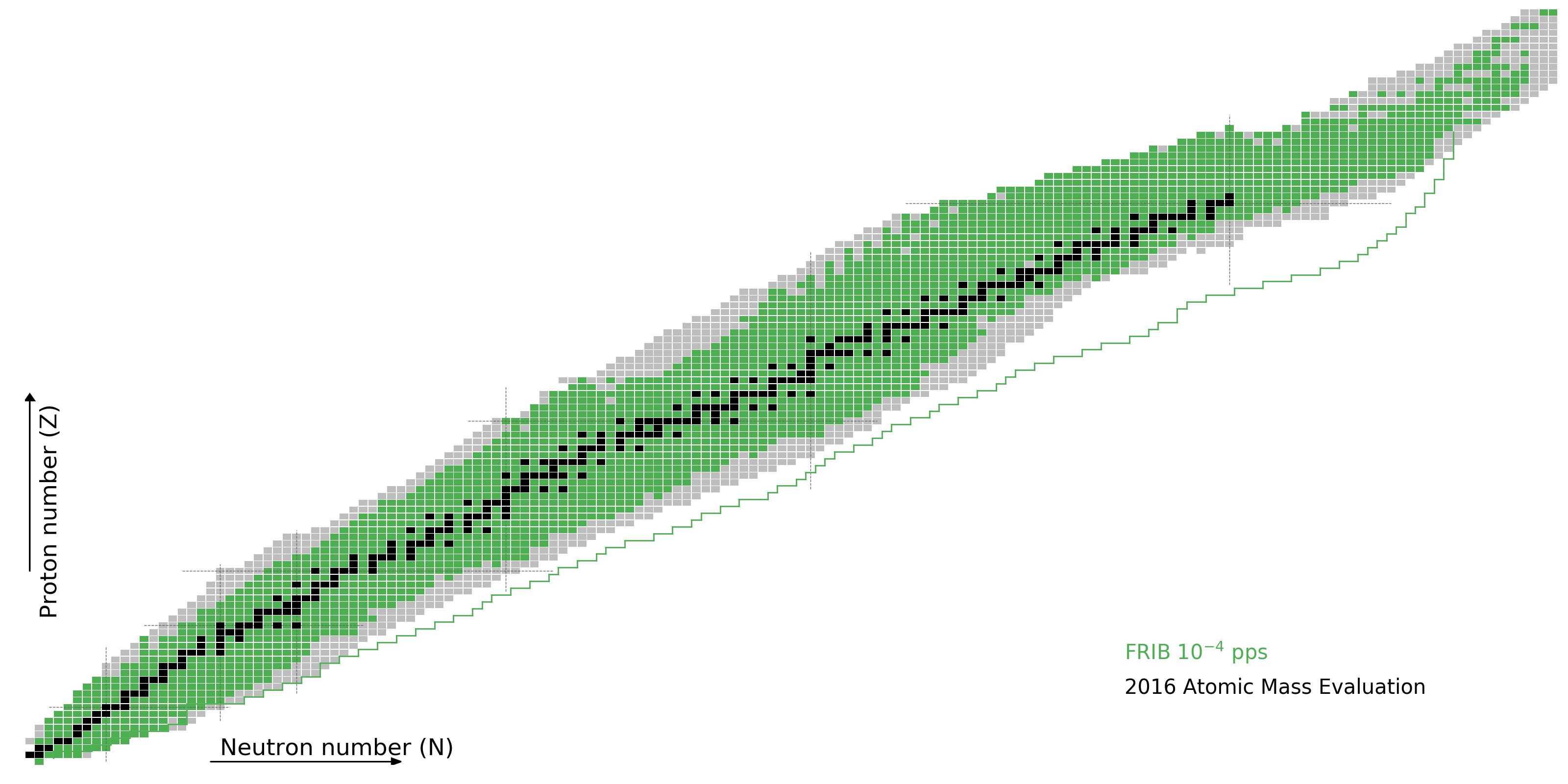Figure by Mumpower

What do we know?

Neutron capture ratesFigure by Mumpower

What do we know?

As of today, to varying degrees of accuracy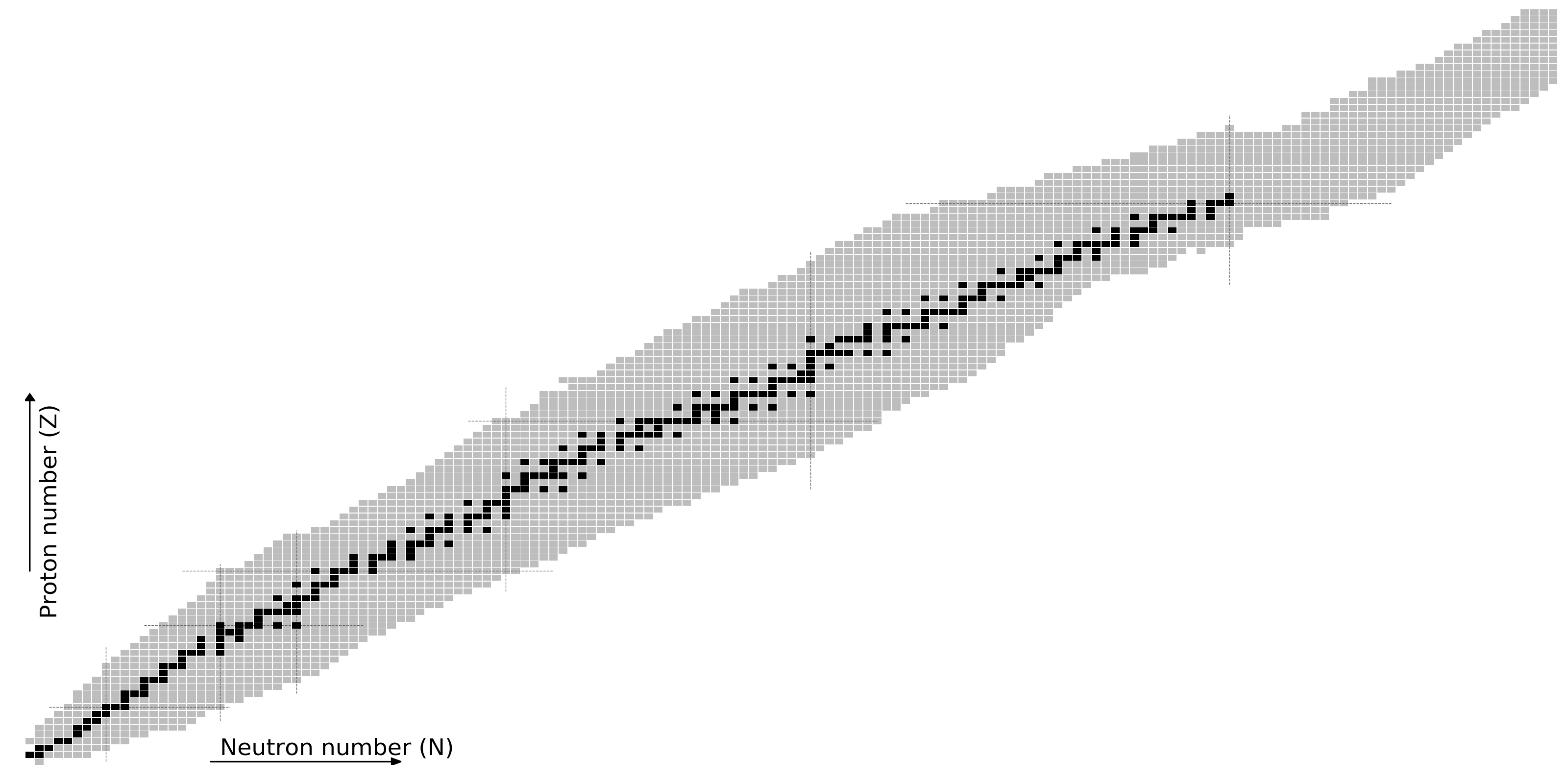Figure by Mumpower

The r-process requires nuclear theory

Even in the era of FRIB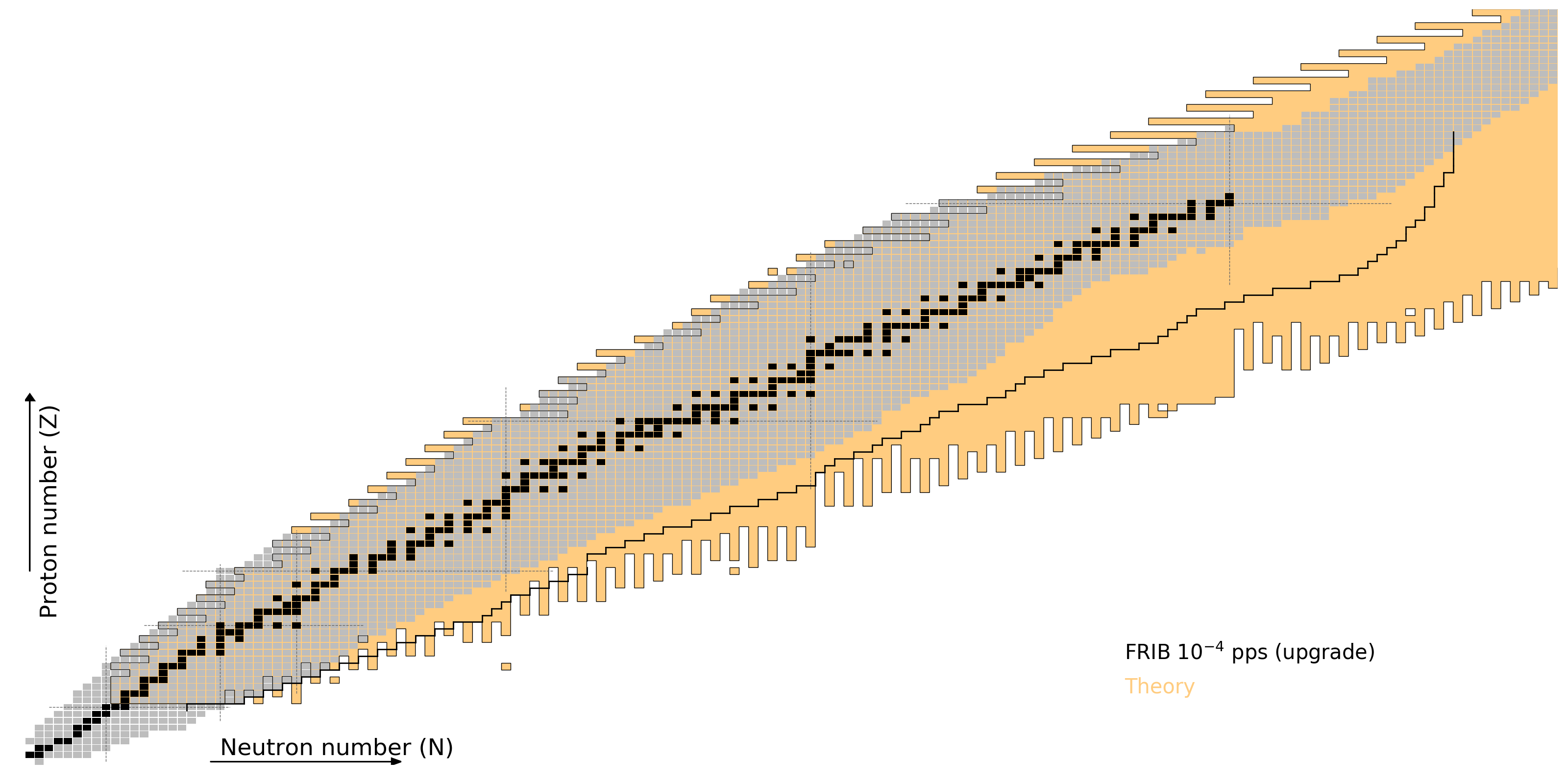Figure by Mumpower

Results

Distribution of $Y_e$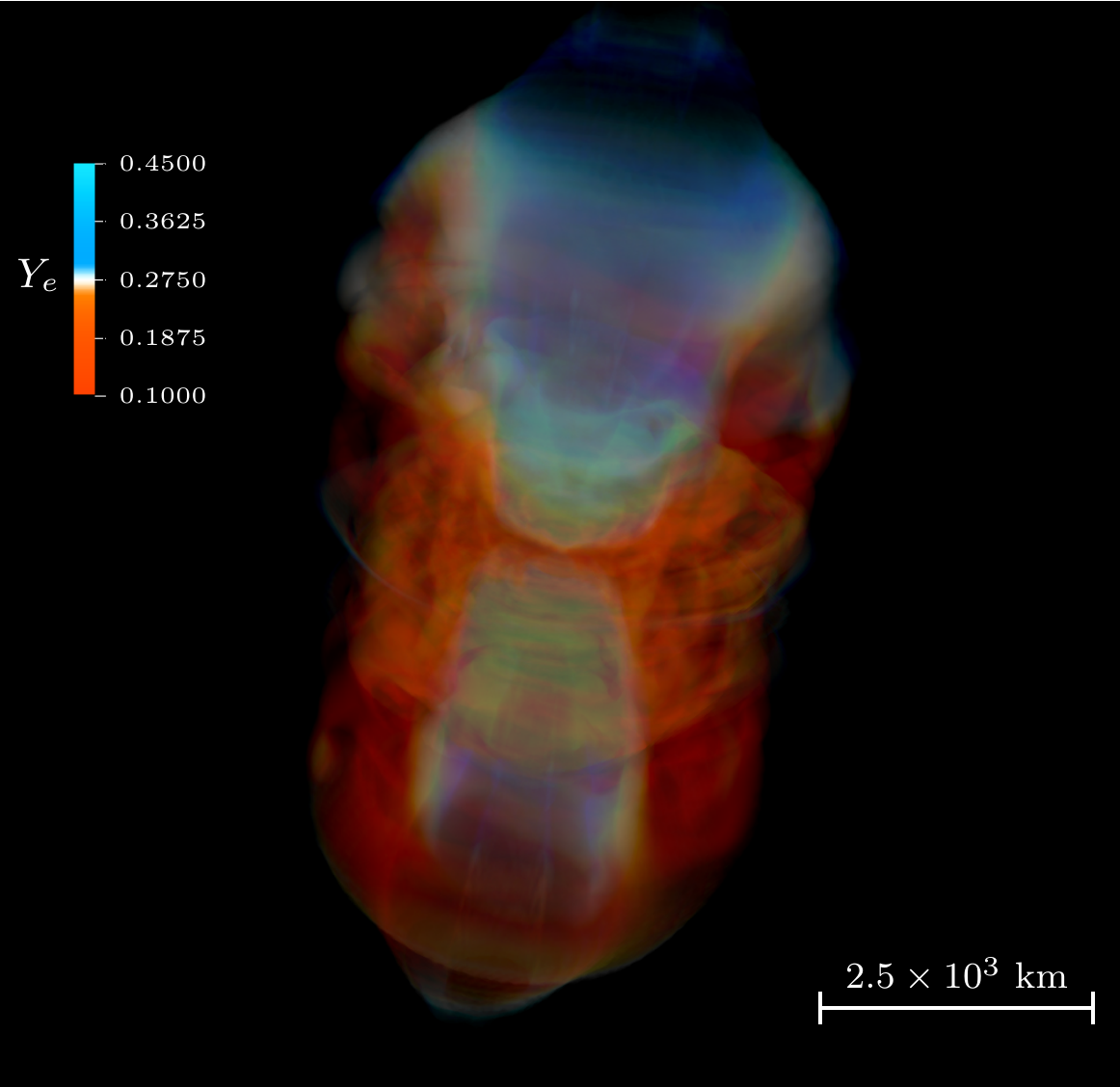Volume rendering of electron fraction, $Y_e$

Jet drives material and voids region near azimuthal axis; high $Y_e$

Miller et al. PRD 100 023008 (2019)

Morphology of ejecta

Miller et al. PRD 100 023008 (2019)

Accretion Disk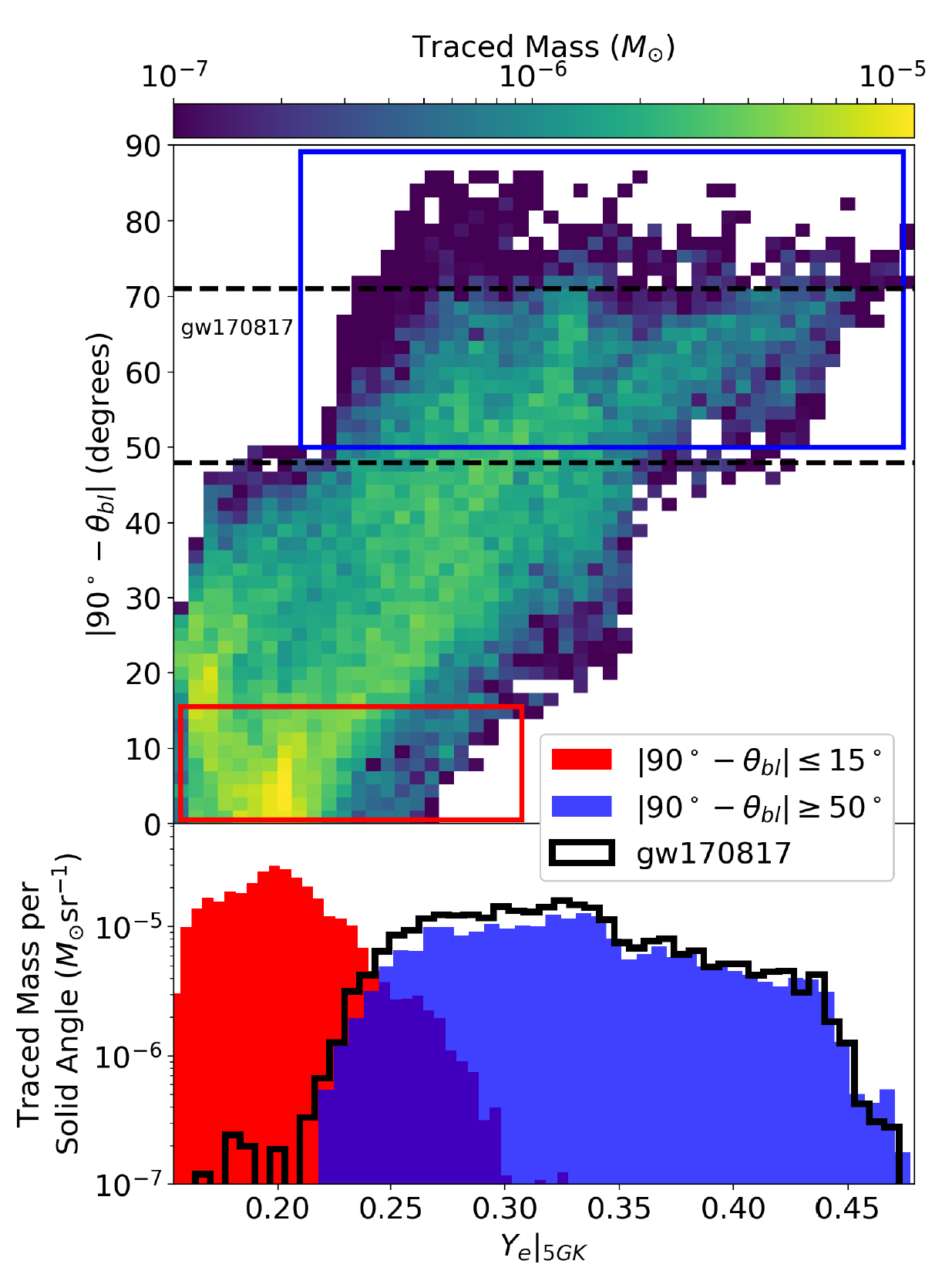Surrounding a black hole

Viewing angle critical for what composition you see

Measured from the mid-plane of the disk

Our model is consistent with observations of a blue kilonova

Red, full $r$-process material seen near mid-plane

The disk can contain a large amount of material

But exactly how much is gravitationally unbound?

Further... What is the important role of neutrinos?

Miller et al. PRD 100 023008 (2019)

Neutrinos impact the nucleosynthesis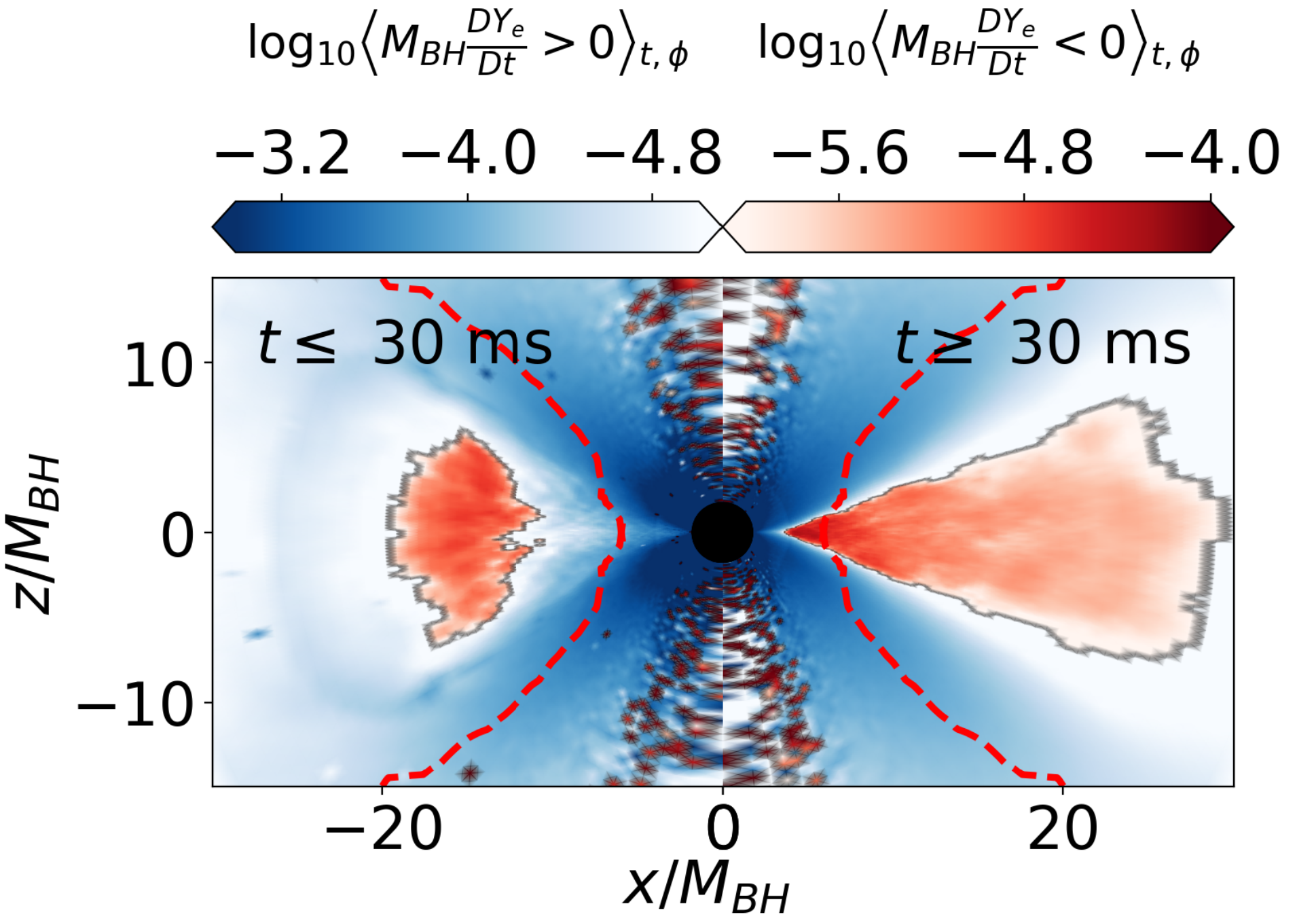Exactly how the ejecta is processed by neutrinos is not fully analyzed yet

Blue region has high $Y_e$; red lower $Y_e$

The transit time away from the disk is crucial

Miller et al. PRD 100 023008 (2019)

Resultant nucleosynthesis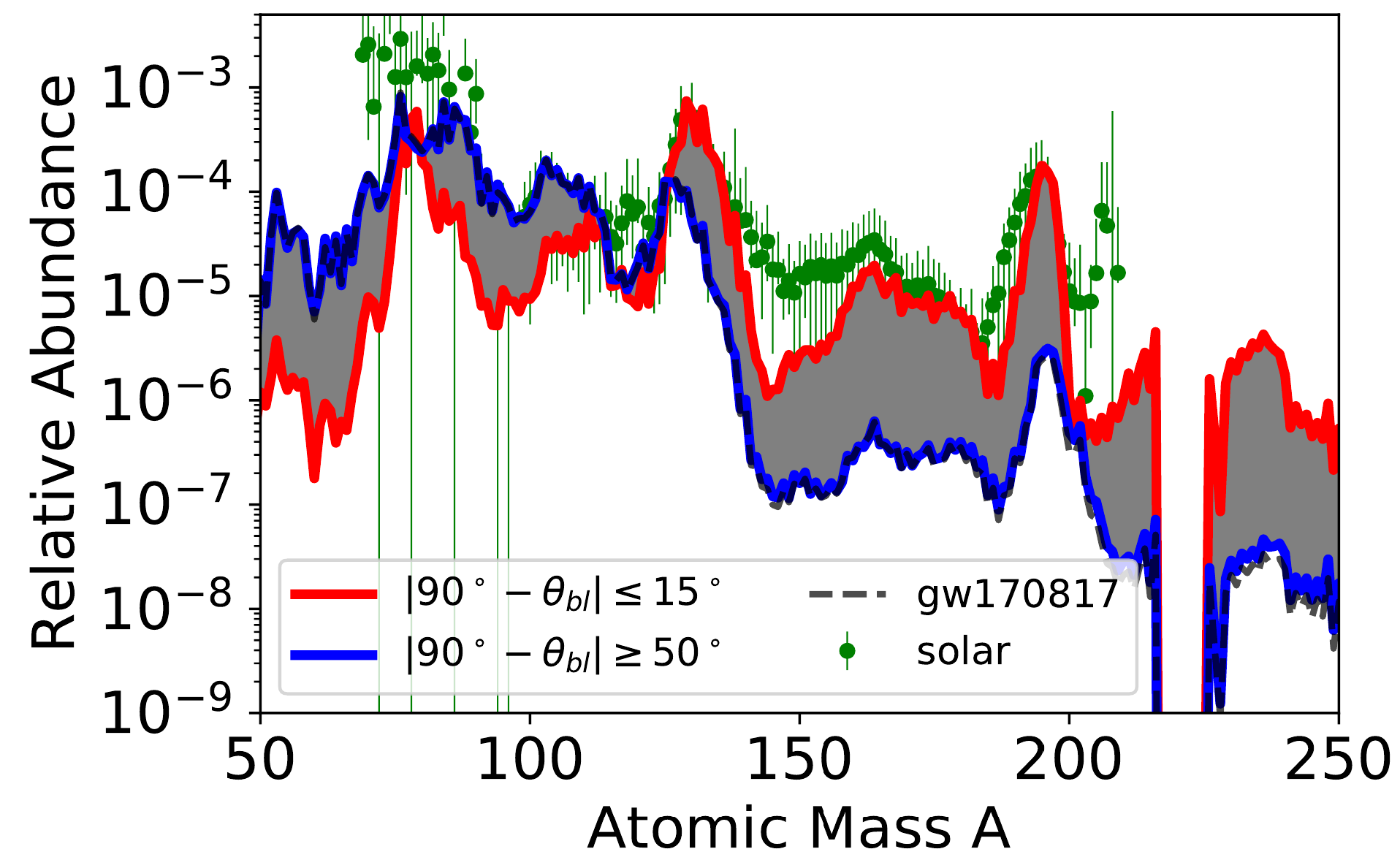Nucleosynthesis simulation with J. Lippuner's SkyNet

Mass weighted trajectories suggest blue kilonova

It is critical to get the full morphology and thereby composition correct...

Miller et al. PRD 100 023008 (2019)

Inferring composition is tricky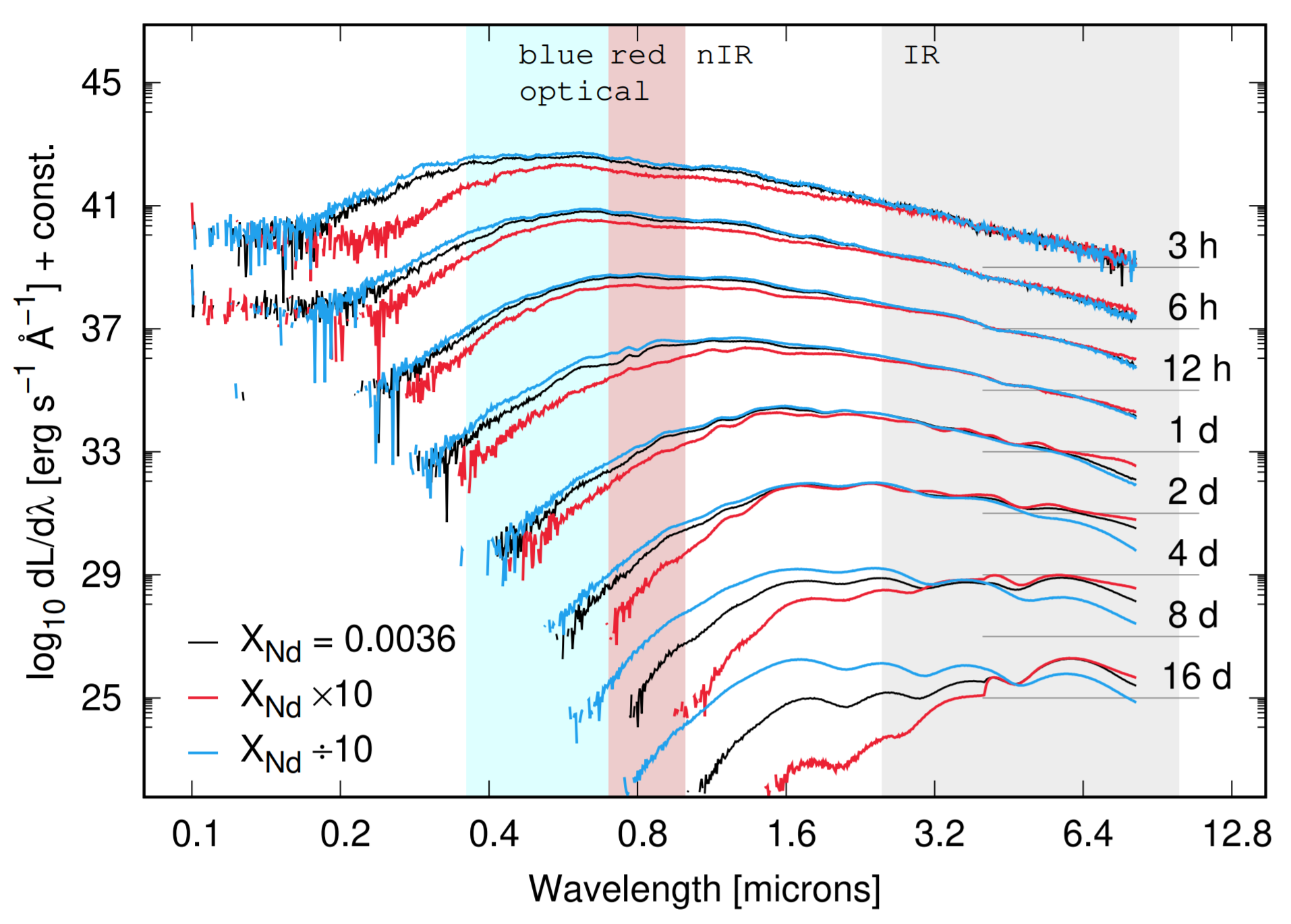One way is to find a proxy in the observed light curve spectrum

Nd is a good choice because it is a high opacity lanthanide

The amount of Nd is absolutely crucial to the observed light curve

But it is important to remember that there are still many astrophysical model degeneracies as this point...

Kasen et al. Nature (2017) • Even et al. submitted (2019)

One way to solve this...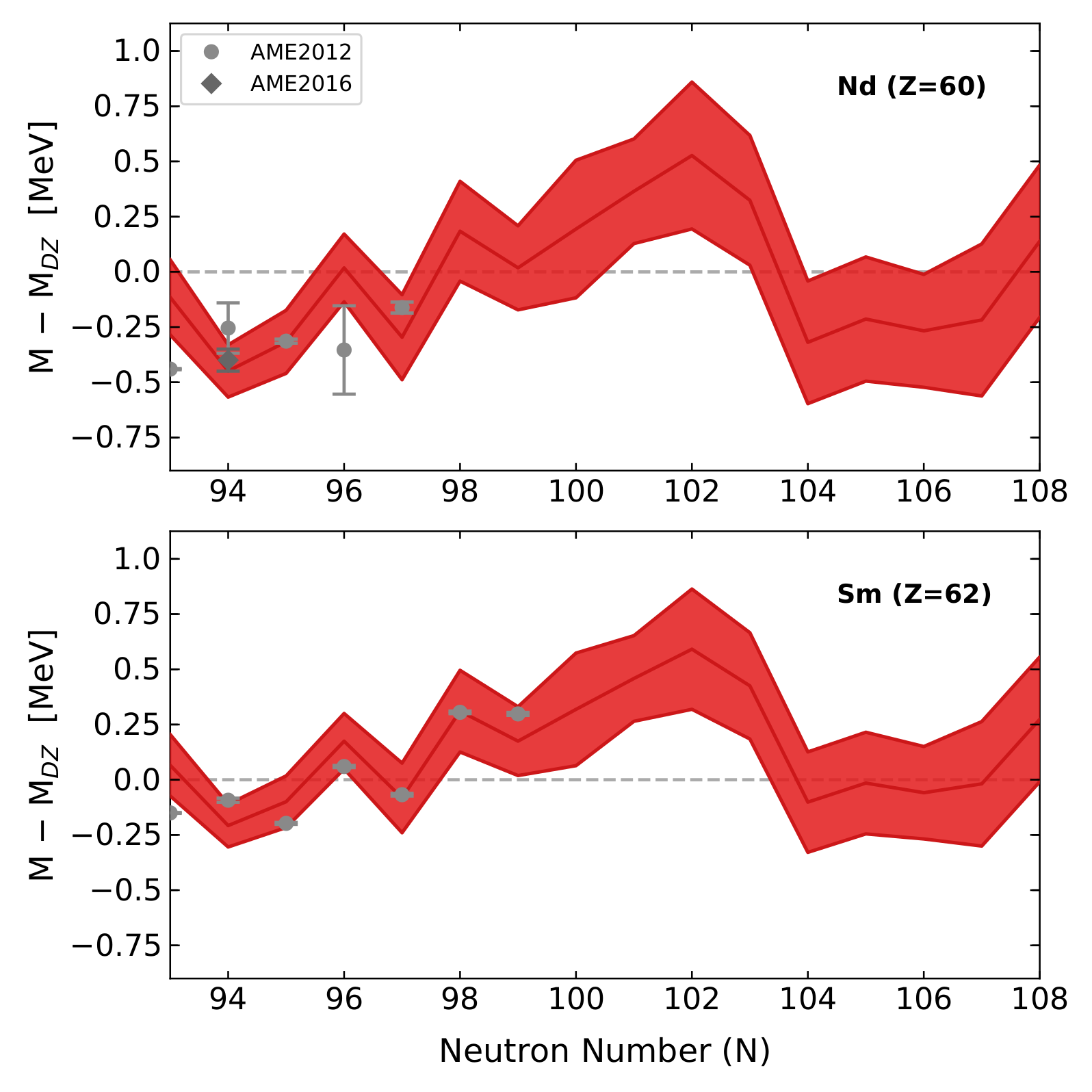Try to better understand Nd production which is also a proxy for the astrophysical conditions

To do this we need to predict the nuclear masses of highly deformed rare earth elements

We use information about the solar isotopic composition to inform our model of nuclear masses

Mumpower et al. ApJ (2012) • Mumpower et al. ApJ (2016) • Mumpower et al. J Phys G (2017) • Orford et al. PRL 120 262702 (2018)

Mass predictions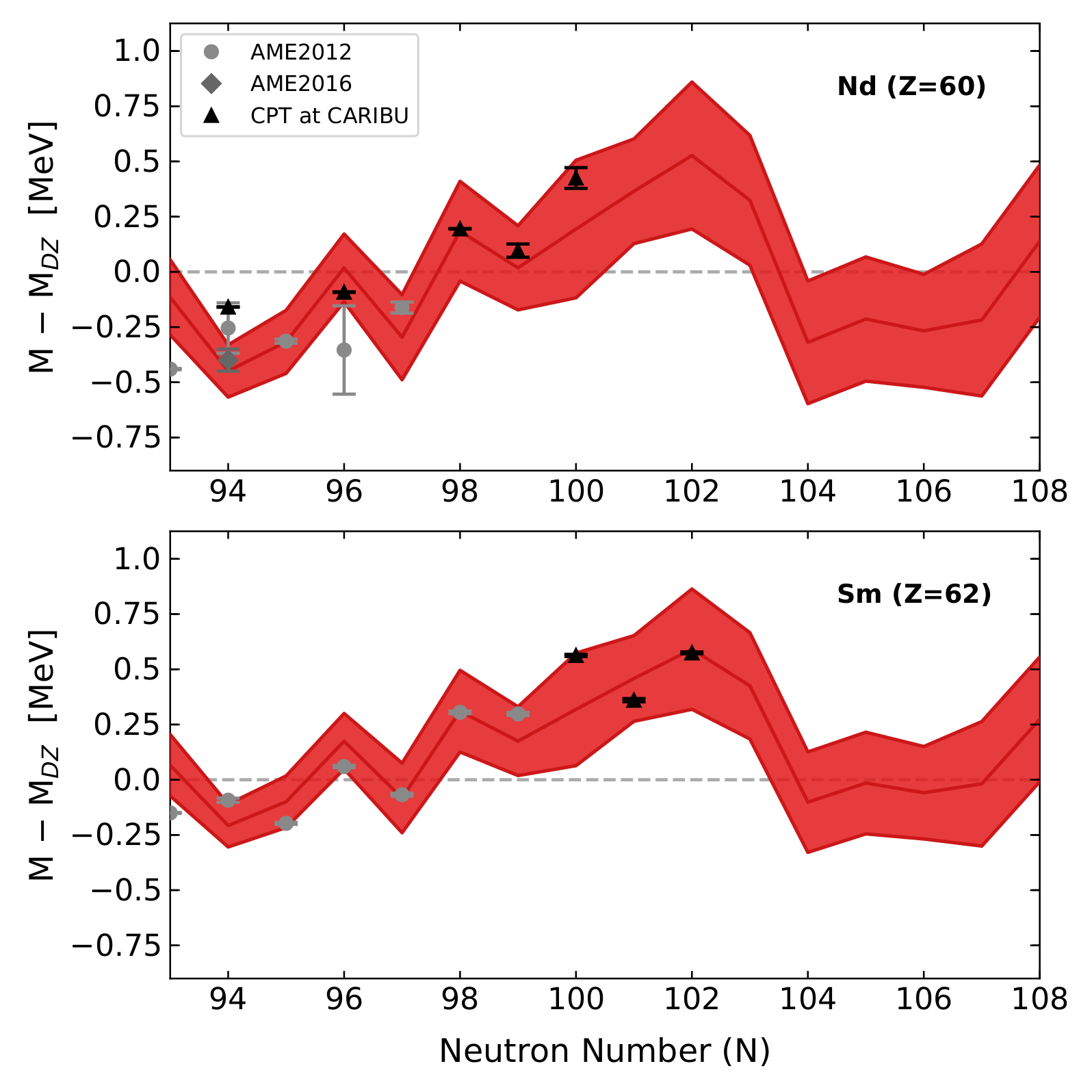The predicted trend matches CPT data!

The downturn between N=102 and N=104 is near the midshell - critical for peak formation - will it also be found?

Orford et al. PRL 120 262702 (2018)

Sensitivity to astrophysical conditions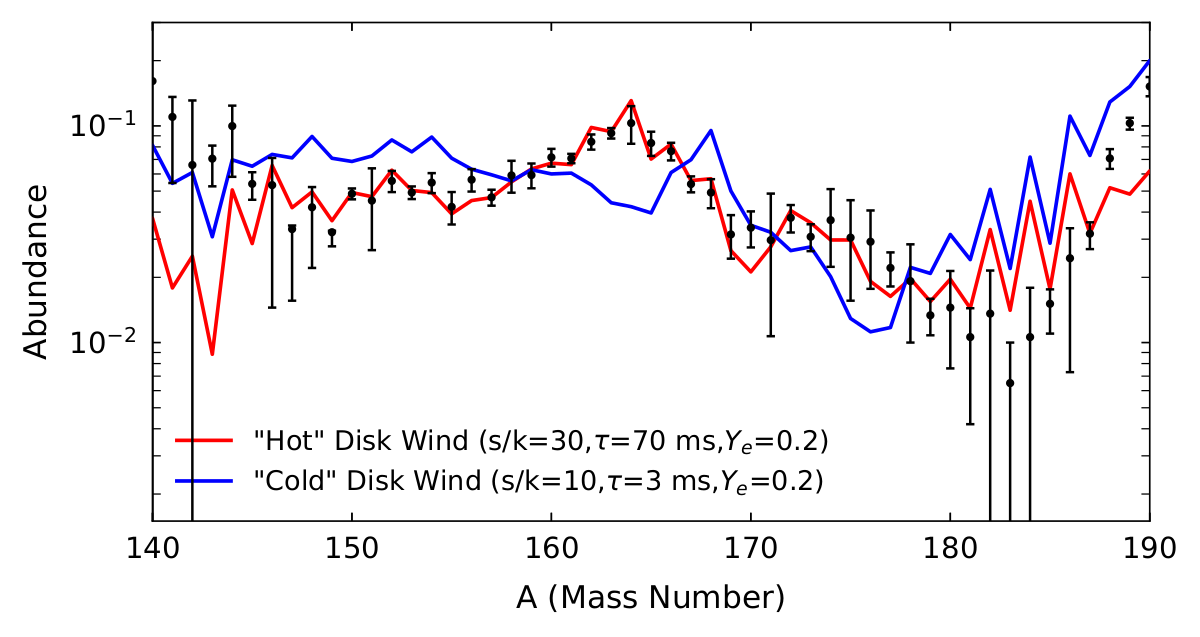The trend is robust for small changes to astrophysical conditions (left)

But if we change the conditions too much (right) we no longer fit the abundances

This result is completely dependent on the N=104 feature which has yet to be measured

The possibility for mass measurements to discrimenate between astrophysical conditions is nearly at hand!

Orford et al. PRL 120 262702 (2018) • Vassh et al. in prep (2019)

The N=126 Factory

Probe nuclei FRIB may have trouble producing...Figure by Mumpower

Importance for the $r$-process

A dirty secret: nucleosynthesis simulations have trouble reproducing
(1) the peak height and (2) the position of the A=195 peak (due to the N=126 shell closure)Final abundances using 20 mass models given the same astro. conditions

The N=126 factory can help us to understand the evolution of shell structure which will greatly impact $r$-process calculations

The N=126 shell closure acts as the gatekeeper to production of the actinides

Calculation by Mumpower

Observational Impact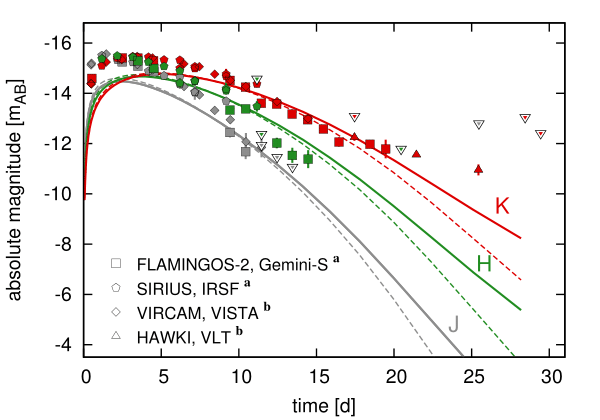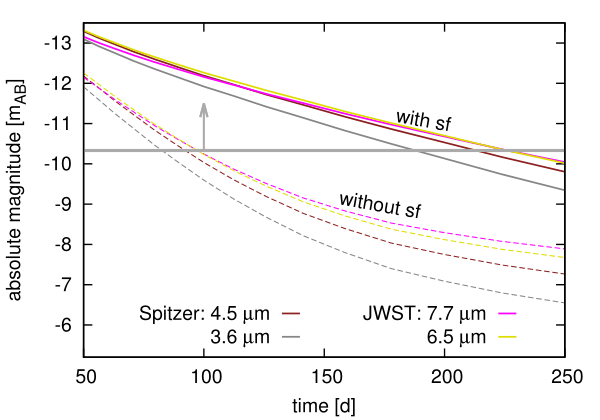Both near- and middle- IR are impacted by the presence of $^{254}$Cf

Late-time epoch brightness can be used as a proxy for actinide nucleosynthesis

Future JWST will be detectable out to 250 days with the presence of $^{254}$Cf

This is all tied to the strength of the N=126 shell closure!

Y. Zhu et al. ApJL 863 2 (2018)

Recently extended actinide studies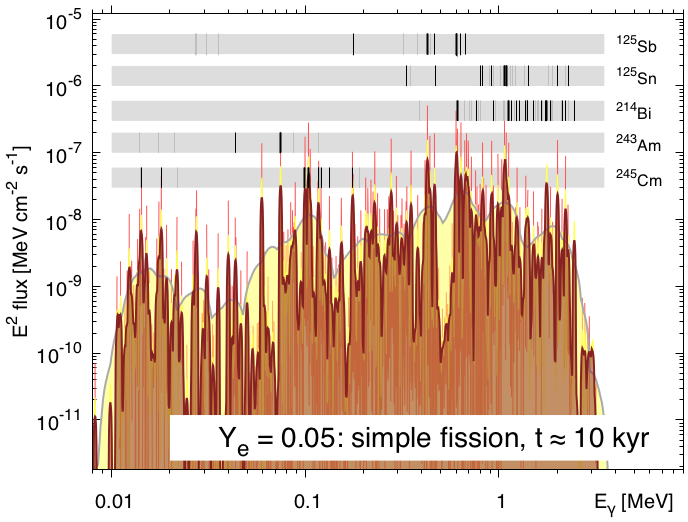Look at the $\gamma$-ray spectrum when producing actinides; event to be very close

Can we do this with future space missions?...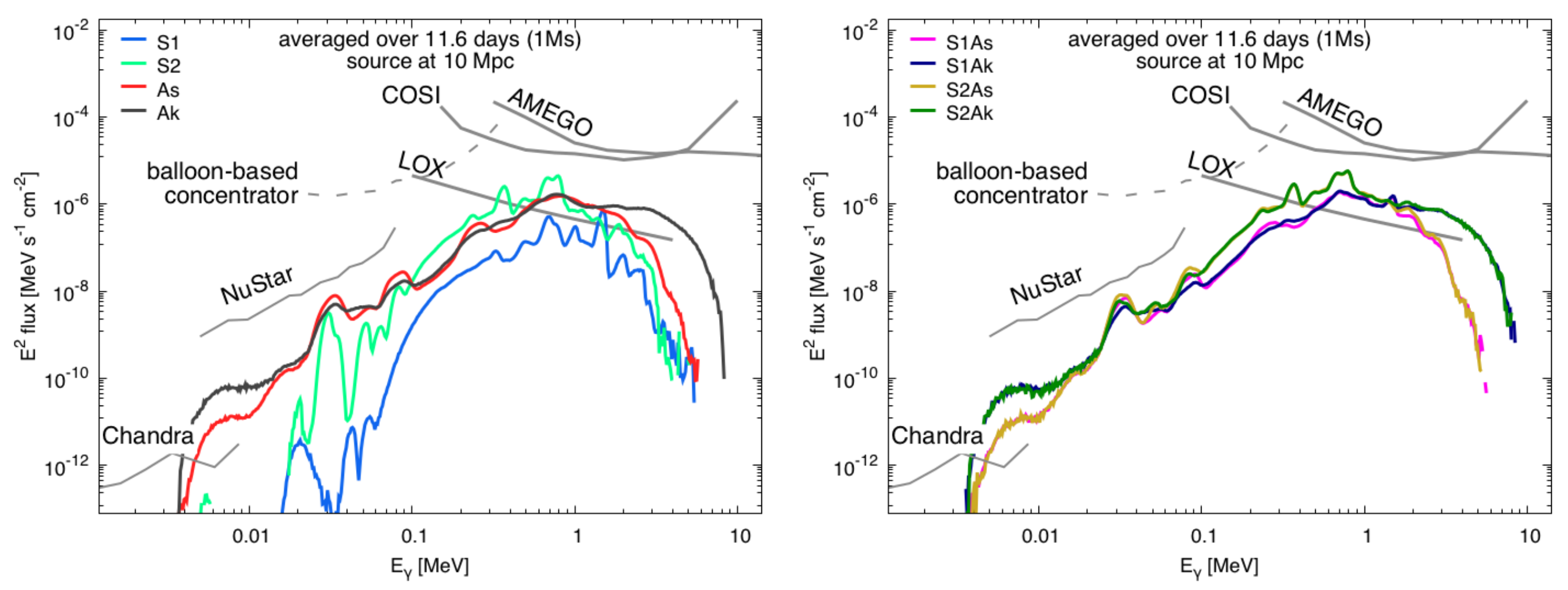Holmbeck et al. ApJ (2018, 2019) • Korobkin et al. submitted ApJ (2019)

Special thanks to

My collaborators

A. Aprahamian, A. Burrows, J. Clark, J. Dolance, W. Even, C. Fontes, C. Fryer, E. Holmbeck, A. Hungerford, P. Jaffke, T. Kawano, O. Korobkin, J. Lippuner, G. C. McLaughlin, J. Miller, W. Misch, P. Möller, R. Orford, G. Savard, T. Sprouse, R. Surman, N. Vassh, M. Verriere, R. Wollaeger, Y. Zhu
& many more...

Student Postdoc CTA Staff FIRE PI

Summary

Our understanding of the $r$-process has been rapidly evolving over the past several years

Recent insights include:

modeling of astrophysical environmentsMulti-messenger observations
Nuclear theory predictionsPrecision experiments

The material from accretion disks may contain the bulk of the ejecta from a binary neutron star merger

Observations depend on angle & morphology & composition

Important if we want to prove definitively that heavy elements such as the actinides were made in an event

Astrophysics can be used as an alternative benchmark for nuclear models, in addition to experimental data

An effort to improve nuclear modeling is ongoing at LANL

Collaboration between Argonne & Los Alamos has been very successful with many projects to come!

Results / Data / Papers @ MatthewMumpower.com